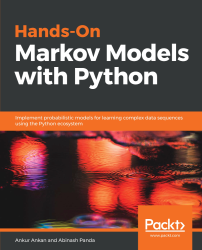•#### Hands-On Markov Models with Python#### Overview of this book

Hidden Markov Model (HMM) is a statistical model based on the Markov chain concept. Hands-On Markov Models with Python helps you get to grips with HMMs and different inference algorithms by working on real-world problems. The hands-on examples explored in the book help you simplify the process flow in machine learning by using Markov model concepts, thereby making it accessible to everyone. Once you’ve covered the basic concepts of Markov chains, you’ll get insights into Markov processes, models, and types with the help of practical examples. After grasping these fundamentals, you’ll move on to learning about the different algorithms used in inferences and applying them in state and parameter inference. In addition to this, you’ll explore the Bayesian approach of inference and learn how to apply it in HMMs. In further chapters, you’ll discover how to use HMMs in time series analysis and natural language processing (NLP) using Python. You’ll also learn to apply HMM to image processing using 2D-HMM to segment images. Finally, you’ll understand how to apply HMM for reinforcement learning (RL) with the help of Q-Learning, and use this technique for single-stock and multi-stock algorithmic trading. By the end of this book, you will have grasped how to build your own Markov and hidden Markov models on complex datasets in order to apply them to projects.
PrefaceFree Chapter
Introduction to the Markov ProcessHidden Markov ModelsState Inference - Predicting the StatesParameter Learning Using Maximum LikelihoodParameter Inference Using the Bayesian ApproachTime Series PredictingNatural Language Processing2D HMM for Image ProcessingMarkov Decision ProcessOther Books You May Enjoy# Reinforcement learning

Reinforcement learning is a different paradigm in machine learning where an agent tries to learn to behave optimally in a defined environment by making decisions/actions and observing the outcome of that decision. So, in the case of reinforcement learning, the agent is not really from some given dataset, but rather, by interacting with the environment, the agent tries to learn by observing the effects of its actions. The environment is defined in such a way that the agent gets rewards if its action gets it closer to the goal.

Humans are known to learn in this way. For example, consider a child in front of a fireplace where the child is the agent and the space around the child is the environment. Now, if the child moves its hand towards the fire, it feels the warmth, which feels good and, in a way, the child (or the agent) is rewarded for the action of moving...